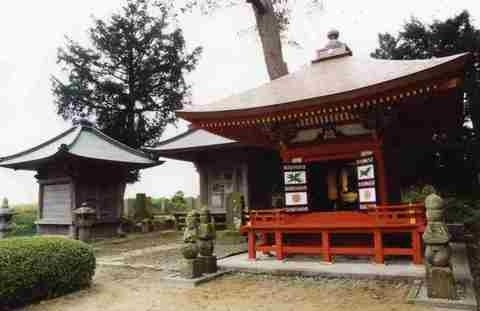查找东北的观光景点

• 选择范围
• 选择地区
• 选择与当前位置的距离

Sort by

• 宮城县

• 宮城县

• 宮城县

• 宮城县

• 宮城县

• 宮城县

• 宮城县

• 宮城县

• 宮城县

• 宮城县

• 宮城县

• 宮城县

• 宮城县

• 宮城县

• 宮城县

• 宮城县

• 宮城县

• 宮城县

• 宮城县

• 宮城县

• 宮城县

• 宮城县

• 宮城县

• 宮城县

• 宮城县

• 宮城县

• 宮城县

• 宮城县

• 宮城县

• 宮城县

• 宮城县

• 宮城县

• 宮城县

• 宮城县

• 宮城县

• 宮城县

The Watari Dates each generation cemetery

• 宮城县The Watari Dates each generation cemetery

• 宮城县

• 宮城县